Updated On : Jul-24,2020 Time Investment : ~10 mins

# Learning Numpy - Simple Tutorial For Beginners - Numpy Matplotlib - Part 21¶

Matplotlib is a plotting library for Python. It is used along with NumPy to provide an environment that is an effective open source alternative for MatLab. It can also be used with graphics toolkits like PyQt and wxPython.

Matplotlib module was first written by John D. Hunter. Since 2012, Michael Droettboom is the principal developer. Currently, Matplotlib ver. 1.5.1 is the stable version available.

Conventionally, the package is imported into the Python script by adding the following statement −

```from matplotlib import pyplot as plt
```

### Example 1¶

```import numpy as np
from matplotlib import pyplot as plt

x = np.arange(1,11)
y = 2 * x + 5
plt.title("Matplotlib demo")
plt.xlabel("x axis caption")
plt.ylabel("y axis caption")
plt.plot(x,y)
plt.show()
```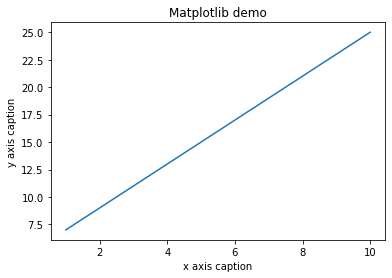Instead of the linear graph, the values can be displayed discretely by adding a format string to the plot() function. Following formatting characters can be used.

Character Description
'-' Dashed line style
'--' Double Dashed line style
'-.' Dash-dot line style
':' Dotted line style
'.' Point marker
',' Pixel marker
'o' Circle marker
'v' Triangle_down marker
'^' Triangle_up marker
'<' Triangle_left marker
'>' Triangle_right marker
'1' Tri_down marker
'2' Tri_up marker
'3' Tri_left marker
'4' Tri_right marker
's' Square marker
'p' Pentagon marker
'*' Star marker
'h' Hexagon1 marker
'H' Hexagon2 marker
'+' Plus marker
'x' X marker
'D' Diamond marker
'd' Thin_diamond marker
**' '** Vline marker
'_' Hline marker
Character Description
'b' Blue
'g' Green
'r' Red
'c' Cyan
'm' Magenta
'y' Yellow
'k' Black
'w' White

### Example 3¶

```import numpy as np
from matplotlib import pyplot as plt

x = np.arange(1,11)
y = 2 * x + 5
plt.title("Matplotlib demo")
plt.xlabel("x axis caption")
plt.ylabel("y axis caption")
plt.plot(x,y,"ob")
plt.show()
```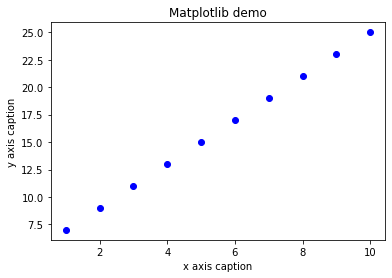## Sine Wave Plot¶

The following script produces the sine wave plot using matplotlib.

```import numpy as np
import matplotlib.pyplot as plt

# Compute the x and y coordinates for points on a sine curve
x = np.arange(0, 3 * np.pi, 0.1)
y = np.sin(x)
plt.title("sine wave form")

# Plot the points using matplotlib
plt.plot(x, y)
plt.show()
```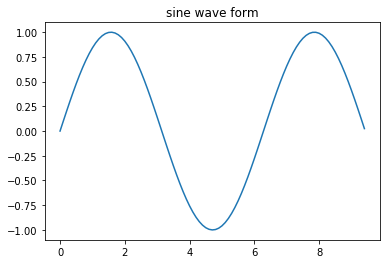## subplot()¶

The subplot() function allows you to plot different things in the same figure. In the following script, sine and cosine values are plotted.

```import numpy as np
import matplotlib.pyplot as plt

# Compute the x and y coordinates for points on sine and cosine curves
x = np.arange(0, 3 * np.pi, 0.1)
y_sin = np.sin(x)
y_cos = np.cos(x)

# Set up a subplot grid that has height 2 and width 1,
# and set the first such subplot as active.
plt.subplot(2, 1, 1)

# Make the first plot
plt.plot(x, y_sin)
plt.title('Sine')

# Set the second subplot as active, and make the second plot.
plt.subplot(2, 1, 2)
plt.plot(x, y_cos)
plt.title('Cosine')

# Show the figure.
plt.show()
```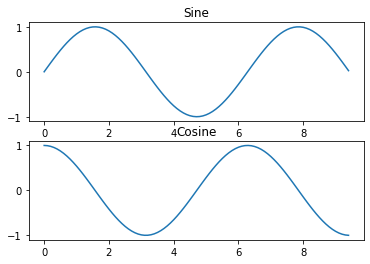## bar()¶

The pyplot submodule provides bar() function to generate bar graphs. The following example produces the bar graph of two sets of x and y arrays.

## Example 4¶

```from matplotlib import pyplot as plt
x = [5,8,10]
y = [12,16,6]

x2 = [6,9,11]
y2 = [6,15,7]
plt.bar(x, y, align = 'center')
plt.bar(x2, y2, color = 'g', align = 'center')
plt.title('Bar graph')
plt.ylabel('Y axis')
plt.xlabel('X axis')

plt.show()
```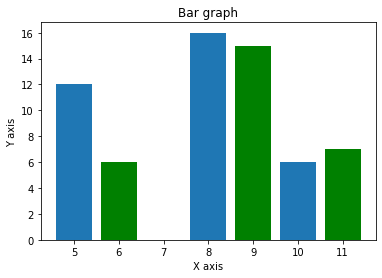Dolly Solanki

## Comfortable Learning through Video Tutorials?

If you are more comfortable learning through video tutorials then we would recommend that you subscribe to our YouTube channel.

## Stuck Somewhere? Need Help with Coding? Have Doubts About the Topic/Code?

When going through coding examples, it's quite common to have doubts and errors.

If you have doubts about some code examples or are stuck somewhere when trying our code, send us an email at coderzcolumn07@gmail.com. We'll help you or point you in the direction where you can find a solution to your problem.

You can even send us a mail if you are trying something new and need guidance regarding coding. We'll try to respond as soon as possible.

## Want to Share Your Views? Have Any Suggestions?

If you want to

• provide some suggestions on topic